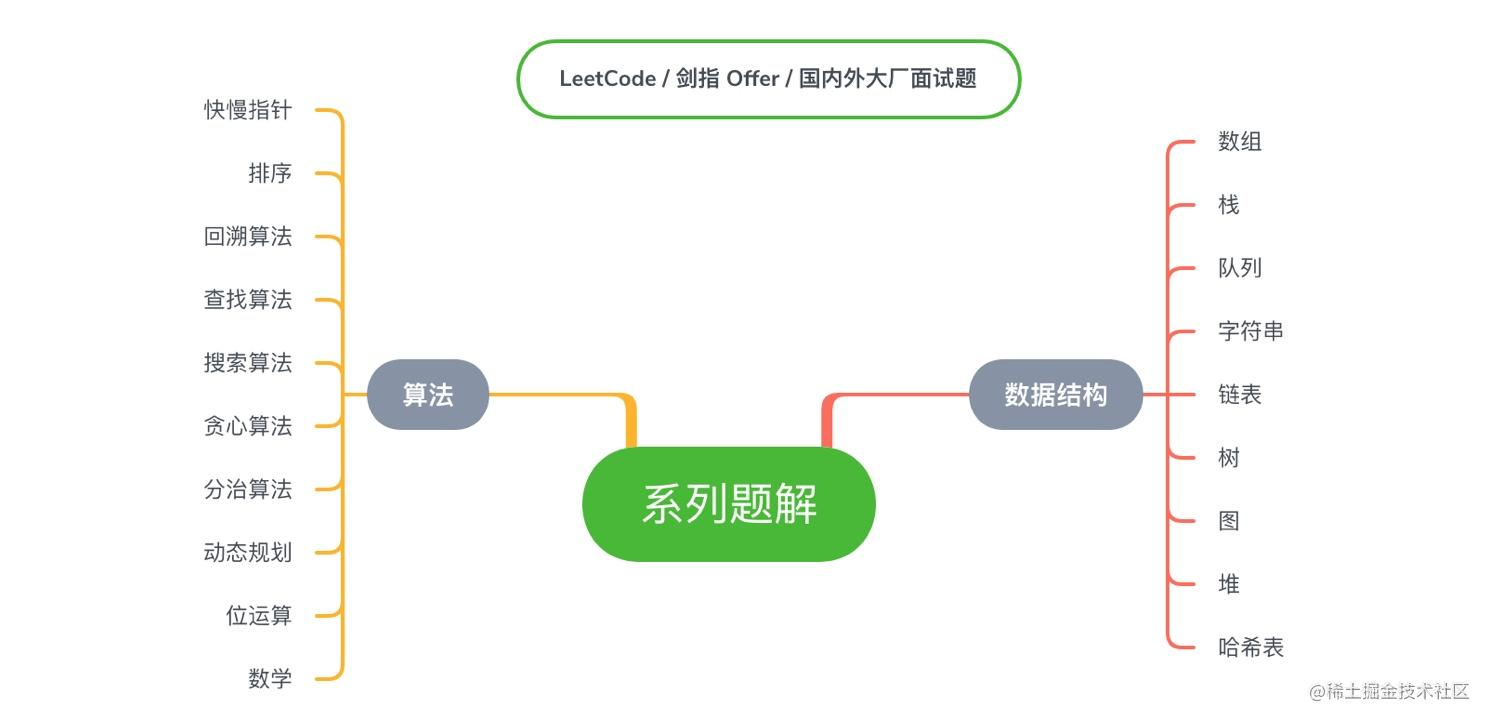## 跟源码学数据结构 | 循环队列

hi 大家好，我是 DHL。公众号：ByteCode ，专注分享最新技术原创文章，涉及 Kotlin、Jetpack、算法动画、数据结构 、系统源码、 LeetCode / 剑指 Offer / 多线程 / 国内外大厂算法题 等等。

• 队列的定义？
• JDK 源码是如何设计队列？
• 队列的大小为什么要设置成 2 的整数次幂？
• 位运算 效率比 非位运算 快多少？
• ArrayDeque 的 2 的整数次幂计算逻辑和 HashMap 有什么区别？
• 自己如何设计一个循环队列？

## 队列的定义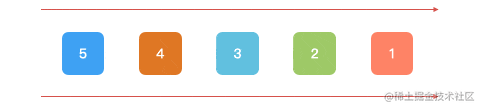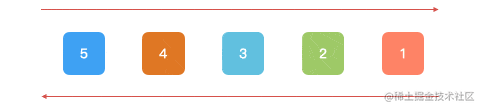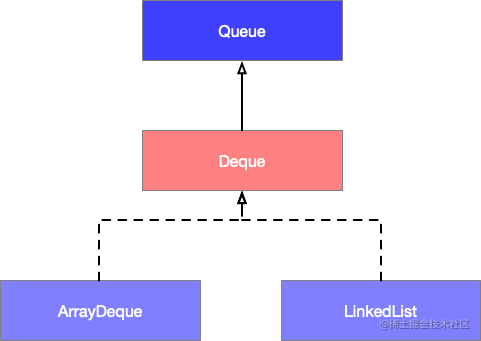## 循环队列

`ArrayDeque``LinkedList` 数据结构的特点如下所示：

ArrqyDeque 循环数组 初始化：16

0(n) 0(1)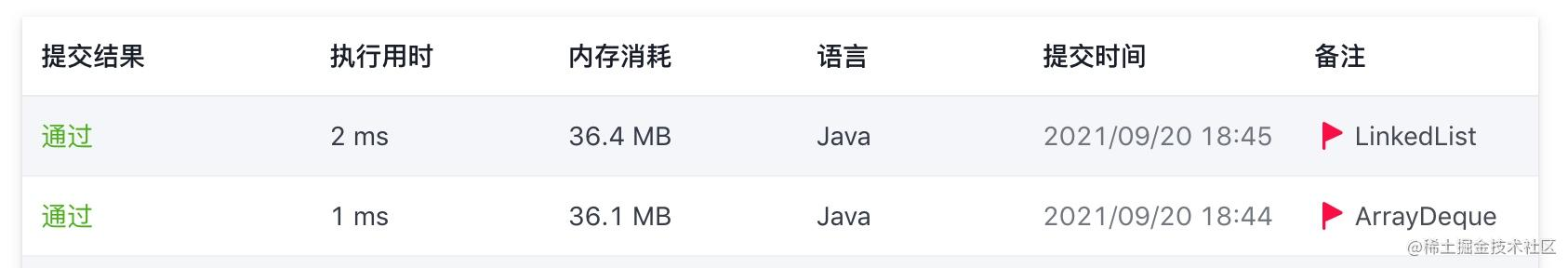### ArrayDeque 源码分析#### 成员变量分析

`ArrayDeque` 内部有四个变量： 数组 `elements` 、 头指针 `head` 、 尾指针 `tail` 、 最小初始化容量 `MIN_INITIAL_CAPACITY`

``````// 数组的长度，总是 2 的整数次幂
transient Object[] elements;
// 头指针，表示队首元素所在位置
// 尾指针，表示队尾元素所在位置
transient int tail;
// 最小初始化容量是 8（JDK 8）， 这是为了保证初始容量都是 2 的整数次幂, 减少计算步骤
private static final int MIN_INITIAL_CAPACITY = 8;

#### 构造方法分析

``````// 无参构造方法，直接初始化容量为 16 的数组
public ArrayDeque() { elements = new Object; }

public ArrayDeque(int numElements) { allocateElements(numElements); }

// 计算数组的大小，返回值是最接近 2 的整数次幂
private void allocateElements(int numElements) {
// 最小初始化容量是 8（JDK 8）
int initialCapacity = MIN_INITIAL_CAPACITY;
if (numElements >= initialCapacity) {
// 大于或者等于初始化容量时
// 会调整为最接近 2 的整数次幂，例如 9 -> 16
initialCapacity = numElements;
initialCapacity |= (initialCapacity >>>  1);
initialCapacity |= (initialCapacity >>>  2);
initialCapacity |= (initialCapacity >>>  4);
initialCapacity |= (initialCapacity >>>  8);
initialCapacity |= (initialCapacity >>> 16);
initialCapacity++;

// 当传人值非常大，经过计算变成负数，会重新初始化为 2 ^ 30
if (initialCapacity < 0)
initialCapacity >>>= 1;
}
elements = new Object[initialCapacity];
}

• 无参构造方法（默认），直接初始化容量为 16 的数组
• 有参构造方法，会初始化最接近 2 的整数次幂的大小的数组。而 `allocateElements(numElements)` 方法的返回值是最接近 2 的整数次幂

``````例如 2^n-1 = 16

int len = 16;
int x1 = 6 & (len -1);
System.out.println("x1 = " + x1); // x1 = 6

x1 = 20 & (len -1);
System.out.println("x1 = " + x1); // x1 = 4

``````例如 2^n-1 = 16

int len = 16;
int x1 = -10 & (len - 1);
System.out.println("x1 = " + x1); // x1 = 6

x1 = 0 & (len - 1);
System.out.println("x1 = " + x1); // x1 = 0

x1 = -20 & (len - 1);
System.out.println("x1 = " + x1); // x1 = 12

`ArrayDeque` 的 2 的整数次幂计算逻辑，相比于 `HashMap` 的计算逻辑是有一点区别的，如下图所示。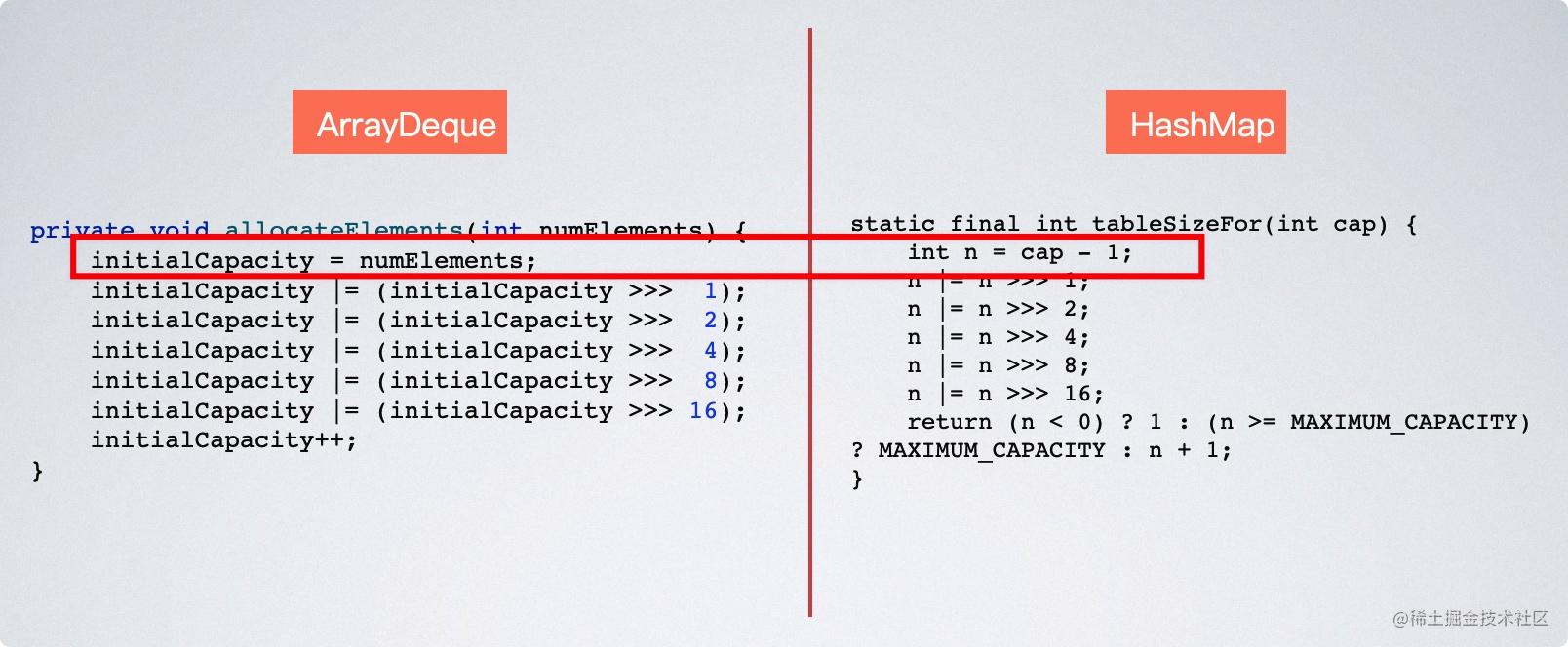`HashMap` 的 2 的整数次幂计算逻辑，在进行位运算之前先执行了 `cap - 1` 的操作，而 `ArrayDeque` 没有，这么做的区别在于，假设传进去来的是 8 正好是 2 的整数次幂， `ArrayDeque` 计算出来的结果是 16，而 `HashMap` 的计算结果是其本身 8 ，这么做的目的，是为了省内存。

#### 元素入队分析

``````......
if (e == null)
throw new NullPointerException();
elements[tail] = e;
if ( (tail = (tail + 1) & (elements.length - 1)) == head)
doubleCapacity(); // 扩容为两倍，通过数组拷贝的方式进行扩容
}

``````(tail = (tail + 1) & (elements.length - 1)) == head

• 重新计算 tail 的索引指向的下一个位置
• 判断队列是否已满，如果已满执行 `doubleCapacity()` 方法进行扩容

``````public boolean isEmpty() {
}

#### 元素出队分析

``````public E pollFirst() {
@SuppressWarnings("unchecked")
E result = (E) elements[h]; // 获取队头元素
// 返回值为 null，表示队列为空，直接返回
if (result == null)
return null;
elements[h] = null;     // 重置当前 head 的位置为空
head = (h + 1) & (elements.length - 1); // 重新计算 head 指向的下一个位置
return result;
}

JDK 之所以这么做是为了提高程序的执行效率，接下来我们通过一个案例来验证 位运算非位运算 它们的执行效率。

## 设计循环队列

• MyCircularQueue(k): 构造器，设置队列长度为 k 。
• Front: 从队首获取元素。如果队列为空，返回 -1 。
• Rear: 获取队尾元素。如果队列为空，返回 -1 。
• enQueue(value): 向循环队列插入一个元素。如果成功插入则返回真。
• deQueue(): 从循环队列中删除一个元素。如果成功删除则返回真。
• isEmpty(): 检查循环队列是否为空。
• isFull(): 检查循环队列是否已满。

``````MyCircularQueue circularQueue = new MyCircularQueue(3); // 设置长度为 3
circularQueue.enQueue(1);  // 返回 true
circularQueue.enQueue(2);  // 返回 true
circularQueue.enQueue(3);  // 返回 true
circularQueue.enQueue(4);  // 返回 false，队列已满
circularQueue.Rear();  // 返回 3
circularQueue.isFull();  // 返回 true
circularQueue.deQueue();  // 返回 true
circularQueue.enQueue(4);  // 返回 true
circularQueue.Rear();  // 返回 4

• 所有的值都在 0 至 1000 的范围内
• 操作数将在 1 至 1000 的范围内
• 请不要使用内置的队列库

### 方法一：位运算

• `data` ：表示固定的数组，用作循环队列，而队列的长度是最接近于 2 的整数次幂
• `allocateElements()` ：用于计算队列的大小，返回值是最接近 2 的整数次幂
• `head` ：表示的队列的头指针索引
• `tail` ：表示队列的尾指针索引
• `count`：表示队列中的元素数量
• `size` ：表示队列中最多可以容纳的元素数量

``````((tail + 1) & (data.length - 1)) == head || (count >= size)

• 任何一个数 和 `2^n-1` 做与运算，保证头指针 `head` 和尾指针 `tail` 的索引，都落在队列范围内
• 队列的长度是最接近于 2 的整数次幂，会大于实际的队列大小，所以需要变量 `count` 统计当前队列中的元素数量，如果 `count >= size` 表示队列已满

``````head == tail

• 时间复杂度：O(1) ，数组存储都是按顺序存放的，具有随机访问的特点
• 空间复杂度：O(N) ，N 为数组的长度

``````class MyCircularQueue {
private int size;
private int tail;
private int[] data;
private int count;

public MyCircularQueue(int k) {
size = k;
data = new int[allocateElements(k)];
}

// 计算队列的大小，返回值是最接近 2 的整数次幂
int allocateElements(int numElements) {
int initialCapacity = numElements;
// 调整为最接近 2 的整数次幂，例如 9 -> 16
initialCapacity |= (initialCapacity >>> 1);
initialCapacity |= (initialCapacity >>> 2);
initialCapacity |= (initialCapacity >>> 4);
initialCapacity |= (initialCapacity >>> 8);
initialCapacity |= (initialCapacity >>> 16);
initialCapacity++;

// 当传人值非常大，经过计算变成负数，会重新初始化为 2 ^ 30
if (initialCapacity < 0)
initialCapacity >>>= 1;
return initialCapacity;
}

public boolean enQueue(int value) {
if (isFull()) {
return false;
}

data[tail] = value;
tail = (tail + 1) & (data.length - 1);
count++;
return true;
}

public boolean deQueue() {
if (isEmpty()) {
return false;
}

count--;
return true;
}

public int Front() {
if (isEmpty()) {
return -1;
}
}

public int Rear() {
if (isEmpty()) {
return -1;
}
// tail - 1 取最近存放的元素
return data[(tail - 1) & (data.length - 1)];
}

public boolean isEmpty() {
}

public boolean isFull() {
return ((tail + 1) & (data.length - 1)) == head || (count >= size);
}
}

### 方法二： 非位运算

• `data` ：表示固定的数组，用作循环队列
• `head` ：表示的队列的头指针
• `tail` ：表示队列的尾指针

``````(tail + 1) % size == head

``````head == tail

• 时间复杂度：O(1) ，数组存储都是按顺序存放的，具有随机访问的特点
• 空间复杂度：O(N)，N 为数组的长度

``````class MyCircularQueue {
int[] data;
int tail;
int size;

public MyCircularQueue(int k) {
// k + 1 有两个原因
// 1. 为了避免冲突循环数组中任何时刻一定至少有一个位置不存放有效元素
// 2. 当  k = 4 ，下标从 0 开始的，假设不移动 head， k 也不 +1 ，第四个元素始终放不进去
size = k + 1;
data = new int[size];
tail = 0;
}

public boolean enQueue(int value) {
if (isFull()) return false;
data[tail] = value;
tail = (tail + 1) % size;
return true;
}

public boolean deQueue() {
if (isEmpty()) return false;
return true;
}

public int Front() {
if (isEmpty()) return -1;
}

public int Rear() {
if (isEmpty()) return -1;
// 因为数组中任何时刻一定至少有一个位置不存放有效元素，所以 tail - 1 取最近存放的元素
// 假设 tail = 0 时，tail - 1 就会变成负数，下标会越界，所以 tail - 1 + size) % size
return data[(tail - 1 + size) % size];
}

public boolean isEmpty() {
}

public boolean isFull() {
return (tail + 1) % size == head;
}
}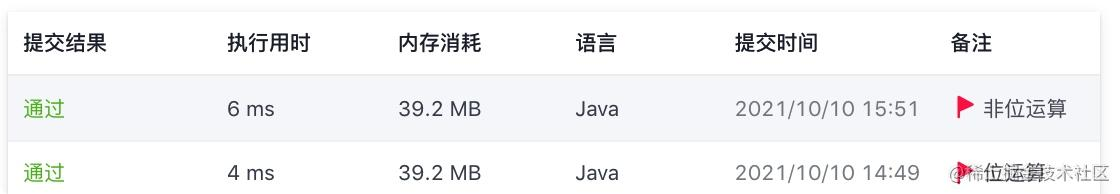• 个人博客，将所有文章进行分类，欢迎前去查看 hi-dhl.com

• KtKit 小巧而实用，用 Kotlin 语言编写的工具库，欢迎前去查看 KtKit

• 计划建立一个最全、最新的 AndroidX Jetpack 相关组件的实战项目 以及 相关组件原理分析文章，正在逐渐增加 Jetpack 新成员，仓库持续更新，欢迎前去查看 AndroidX-Jetpack-Practice

• LeetCode / 剑指 offer / 国内外大厂面试题 / 多线程 题解，语言 Java 和 kotlin，包含多种解法、解题思路、时间复杂度、空间复杂度分析